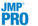Predictive and Specialized Modeling > Support Vector Machines > Overview of Support Vector Machines
Publication date: 10/01/2019

#Overview of Support Vector Machines

A support vector machine (SVM) model is a supervised learning algorithm that is used to classify binary and categorical response data. A model is fit on a set of training data where the responses are known. Then, the model is used to predict the categories of new observations.

SVM models classify data by optimizing a hyperplane that separates the classes. This can also be viewed as finding the hyperplane that maximizes the margin between the classes. In simple problems, this hyperplane is linear. However, more complicated data often cannot be separated linearly. For these scenarios, the SVM platform provides the option to use a radial basis function kernel to map the points to a nonlinear dimension that can make the classes easier to separate.

The maximization in SVM algorithms is performed by solving a quadratic programming problem. In JMP Pro, the algorithm used by the SVM platform is based on the Sequential Minimal Optimization (SMO) algorithm introduced by John Platt in 1998. Typically, the SVM quadratic programming problem is very large. The SMO algorithm splits the overall quadratic programming problem into a series of smaller quadratic programming problems. Smaller quadratic programming problems are solved analytically instead of numerically, meaning they produce closed-form solutions. Therefore, the SMO algorithm is more efficient than solving the overall quadratic programming problem (Platt 1998).